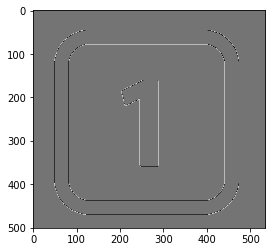## 卷積是什麼？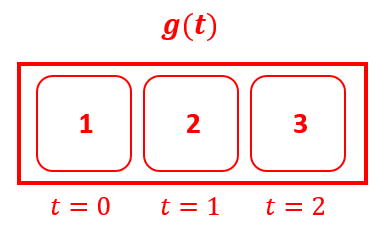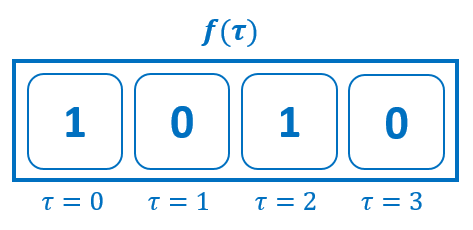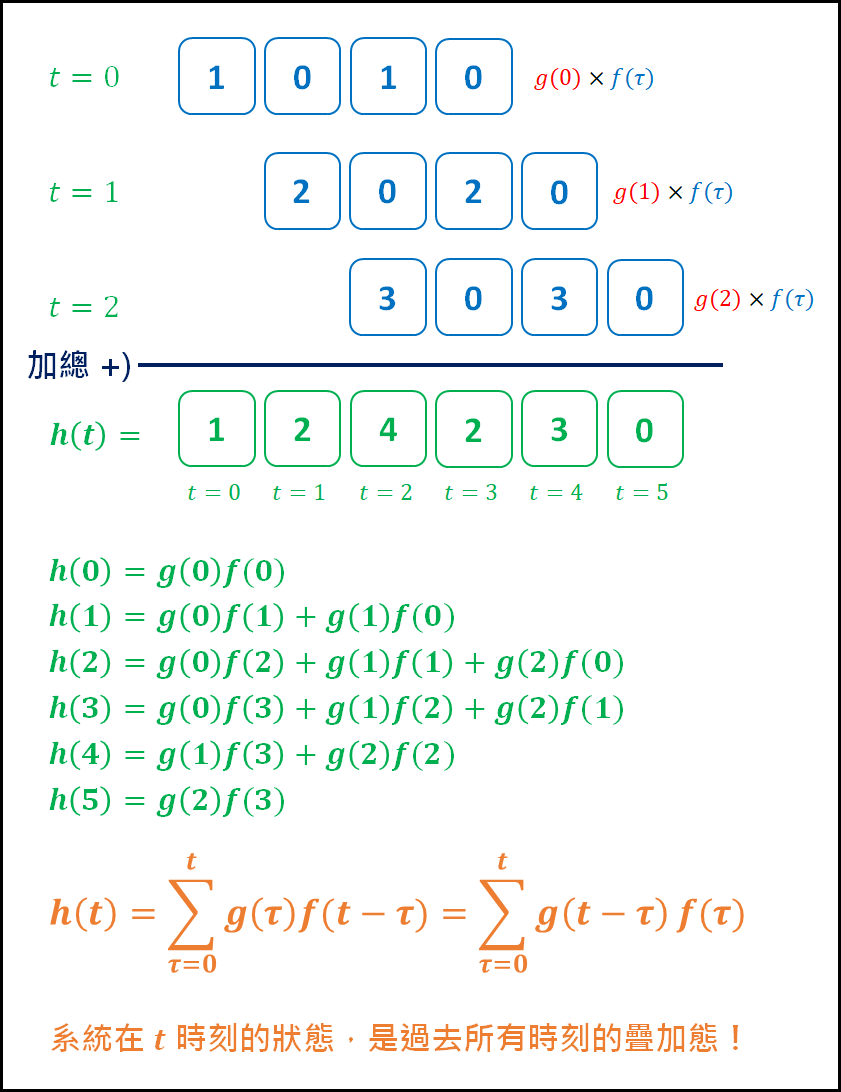#### 看到這邊，我們就能下一個結論： 所謂的「卷積」，也就是做一個「滑動、相乘再相加」的動作。

g 和 f 可以就兩個一維座標軸，組成一個 XY 平面。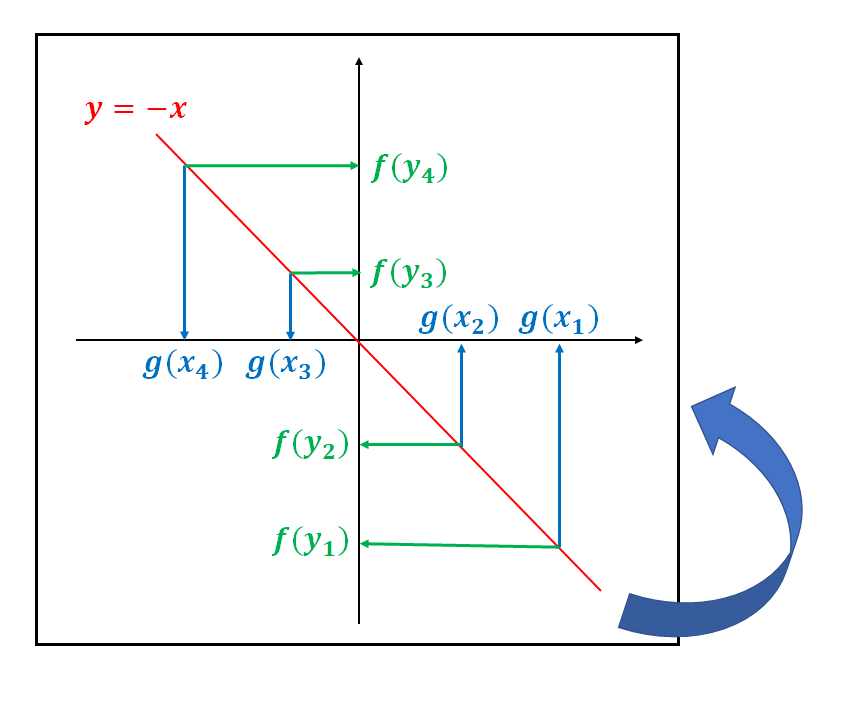τ 和 (t-τ) 就是翻轉180度的意思，畫成圖會更容易理解。## 卷積怎麼用？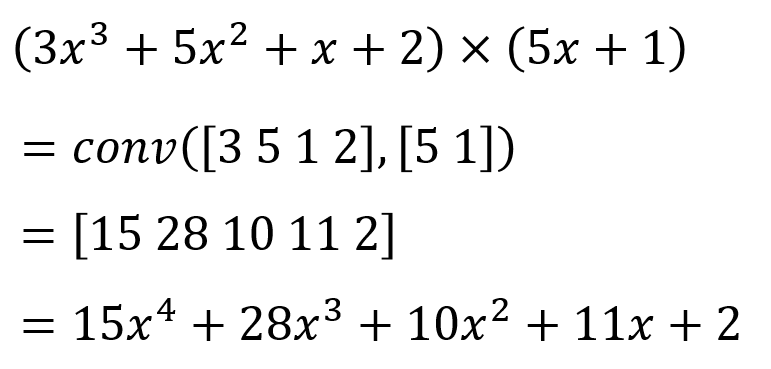length(U) + length(V) - 1

Img1 = conv2( Img, [1, -1], 'same' );
Img1 = abs(Img1);
imshow(Img1)## Tensorflow 的卷積函數Input image size = [N_batch, 5, 5, 3]
filter size = [3, 3, 3, 2]
strides = [1, 2, 2, 1]

tf.nn.convolution
tf.nn.conv1d
tf.nn.conv2d
tf.nn.conv3d ...

tf.nn.conv2d(input, filter, strides, padding,
use_cudnn_on_gpu=True,
data_format='NHWC',
dilations=[1, 1, 1, 1],
name=None )

#### 1. input & data_format：

batch：  圖片數量
height： 圖片高度
width：   圖片寬度
channels：圖片通道數，例如 RGB 影像 channels = 3；灰階影像 channels = 1。

#### 2. filter：

filter_height：   卷積核的長度
filter_width：    卷積核的寬度
in_channels：  輸入圖片的通道數
out_channels：輸出圖片的通道數

#### 3. strides：

" VALID " = 卷積時，不做任何填充，最右邊 column 與最下面 row 會被丟棄。對於 " VALID " 輸出的形狀是 ceil[ (I+F-1)/S ]，也就是 input 的長度 + filter 的長度 - 1 再除以 Stride 值，最後無條件進位。

inputs:         1  2  3  4  5  6  7  8  9  10 11 (12 13)
|________________|                dropped
|_________________|

" SAME " = 嘗試向左和向右均勻填充，但如果要添加的列數是奇數，它將向右添加額外的列，如本例中的情況。對於 "SAME " 輸出的形狀是 ceil[  I / S ]，也就是 input 的長度除以 Stride 值，最後無條件進位。

            pad|                                      |pad
inputs:      0 |1  2  3  4  5  6  7  8  9  10 11 12 13|0  0
|________________|
|_________________|
|________________|

#### 6. dilations：

filter = [ 1, 1, 1,
1, 1, 1,
1, 1, 1 ]

filter = [ 1, 0, 1, 0, 1,
0, 0, 0, 0, 0,
1, 0, 1, 0, 1,
0, 0, 0, 0, 0,
1, 0, 1, 0, 1, ]

## 小結

【Python】TensorFlow學習筆記(七)：卷積深深深幾許

## 附錄

# -*- coding: utf-8 -*-
"""

2018.12.26 Shayne
"""

import cv2
import numpy as np
import tensorflow as tf
import matplotlib.pyplot as plt

# 建立卷積核：高通濾波器
temp = np.array([ [-1, -1, -1],
[-1,  8, -1],
[-1, -1, -1] ], dtype='float32')

# 轉成 tf.nn.conv2d 所須格式
kernal = tf.reshape(tf.Variable(temp), [3, 3, 1, 1])
print(kernal) 
>>> Tensor("Reshape_1:0", shape=(3, 3, 1, 1), dtype=float32)

# 載入灰階影像，並二值化
_, I2 = cv2.threshold(I1, 0, 255, cv2.THRESH_OTSU)

# 轉 uint8 格式成 float32
I2 = I2.astype('float32')

# 轉換成卷積輸入模式
x_img = tf.reshape(I2, [-1, I2.shape, I2.shape, 1])

plt.imshow(I2, cmap='gray')
plt.show()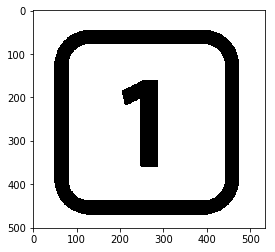# 卷積運算子
y_conv = tf.nn.conv2d(x_img, kernal, strides=[1, 1, 1, 1], padding='SAME')

# 啟動計算圖
with tf.Session() as sess:

# 變數需要初始化
sess.run( tf.global_variables_initializer() )

# 執行運算子
result = sess.run(y_conv)

# 展示結果
I3 = tf.reshape(result, [I2.shape, I2.shape])
I3 = I3.eval()

plt.imshow(I3, cmap='gray')
plt.show()